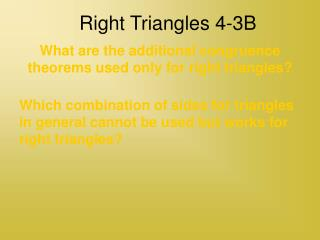Download PresentationRight Triangles 4-3B

# Right Triangles 4-3B

Download Presentation## Right Triangles 4-3B

- - - - - - - - - - - - - - - - - - - - - - - - - - - E N D - - - - - - - - - - - - - - - - - - - - - - - - - - -
##### Presentation Transcript

1. Right Triangles 4-3B What are the additional congruence theorems used only for right triangles? Which combination of sides for triangles in general cannot be used but works for right triangles?

2. If you need to prove that two right triangles are congruent, you can use any of the triangle congruence postulates. However, there are some additional postulates just for right triangles which are special cases for what you already know.

3. F A H G B C Leg-Leg Congruence Theorem (LL) If the legs of a right triangle are congruent to the legs of another right triangle, then the two triangles are congruent. (Same as SAS)

4. H R J I T S Hypotenuse Acute Angle Congruence Theorem (HA) If the hypotenuse and an acute angle of one right triangle are congruent to the hypotenuse and an acute angle of another right triangle, then the two triangles are congruent. (SAA)

5. S E F A R T W X D B C Y Leg Acute Angle Congruence Theorem (LA) If one leg and one acute angle of a right triangle are congruent to the corresponding legs and acute angle of another right triangle, then the two triangles are congruent. (ASA)

6. A M C B O N Hypotenuse Leg Congruence Theorem (HL) If the hypotenuse and a leg of one right triangle are congruent to the hypotenuse and a leg of another right triangle, then the two triangles are congruent.

7. S X X R T S R T R T Proof Statements • Reasons • Given • Per lines form rt angles • Def rt ∆ • Given • Reflexive • HL

8. What are the additional congruence theorems used only for right triangles? • Leg-Leg (LL) • Hypotenuse-Angle (HA) • Leg-Angle (LA) • Hypotenuse-Leg (HL) • Which combination of sides for triangles in general cannot be used but works for right triangles? • Hypotenuse-Leg (HL)

9. Assignment 4-3B Page 295, 1-9, 15, 19